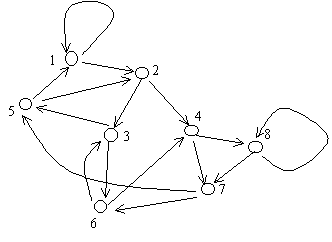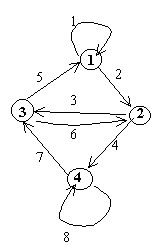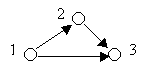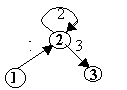Имя: Пароль: Зарегистрироваться Восстановить пароль
03/10/2023 03:20:37

#Рабочее место участника

##Задачи

1916. Transformation of the Graph

Ограничения: время – 1s/2s, память – 32MiB Ввод: input.txt или стандартный ввод Вывод: output.txt или стандартный выводПослать решение Blockly Посылки Темы Где Обсудить (0)

Let find the path going through all the vertices of the oriented graph one time, i.e. find Hamiltonian path. This problem belongs to the class of NP-hard problems, and its straightforward solution needs many hours of calculations. Sometimes it's possible to build new graph G', which contains as mush arcs, as many vertices are in source graph G, and there is bijection φ (one-to-one correspondence) between the set of the vertices of G and the set of the arcs of G' such that if there is an arc (x\ y) in G, where x≠y, then the end of the arc φ(x) in G' coincides with the beginning of the arc φ(y). The bijection φ maps adjacent vertices in G to adjacent arcs in G' and nonadjacent vertices in G to nonadjacent arcs in G'. In this case the problem of finding Hamiltonian path in source graph reduces to the problem of finding Eulerian path in new graph, the last one is much easier. The self-adjacency of vertices in the source graph and arcs in the new graph should not be taken into account, as it has not effect to path searching.
Write the program, which checks the possibility of mentioned transformation for given oriented graph and build new oriented graph.
Input
The first line of the input file contains two integers K and N (1\ ≤\ K\ ≤\ 100, 1\ ≤\ N\ ≤\ K^2), separated by space, where K is quantity of vertices and N is quantity of arcs of source graph G. The following N lines contain pairs of integers from 1 to K, separated by space; each pair describes the beginning and the end of an arc.
Output
The output file contains zero in case of impossibility of mentioned transformation. Else the first line contains the quantity M of vertices of new graph and the following K lines contain information about arcs of new graph; you should write in i-th line the numbers of beginning and end of i-th arc, which corresponds to i-th vertex of source graph. Use arbitrary indexing from 1 up to M for vertices of new graph.

Sample Input #1

8 16
1 1
1 2
2 4
2 3
3 6
3 5
4 8
4 7
6 3
6 4
5 1
5 2
7 6
7 5
8 8
8 7Sample Output #1

4
1 1
1 2
2 3
2 4
3 1
3 2
4 3
4 4Sample Input #2

3 3
1 2
1 3
2 3Sample Output #2

3
1 2
2 2
2 3